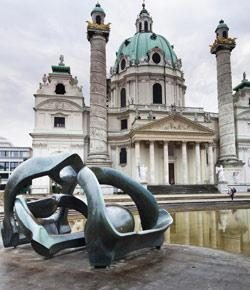# Fs Austria Quiz 1

14 Questions | Attempts: 1198
ShareSettings• 1.
Q1 To prevent vehicle roll during cornering the roll center has to be:
• A.

At 2/3 of the vehicle CoG height

• B.

At the level of the vehicle CoG

• C.

At 1/3 of the unsprung mass CoG height

• D.

At the level of the sprung mass CoG

• E.

• F.

• 2.
With "Pro-Ackermann" Steering the steering angle is bigger on the outer wheel.
• A.

True

• B.

False

• C.

Not related with the steering handle

• D.

Option 4

• 3.
Choose the correct option of penalties for the late submission of following documents:- 1.electronic copy  2. business logic case  3.engineering design report   4.electronic safety form  a. -10 points/day,max  -100 points   b.-10 points/day,max -80 points  c. -10 points/day,max -50  d. -10 points/day,max -70 points
• A.

1-a,2-c,3-b,4-d

• B.

1-a,2-b,3-c,4-d

• C.

1-b,2-c,3-a,4-d

• D.

1-c,2-d,3-b,4-a

• E.

1-d,2-b,3-a,4-c

• 4.
What is the area of the technical inspection sticker that should be left on the upper nose of the vehicle.
• A.

25.4cmX20.3cm

• B.

7.5cmX15cm

• C.

25.4cmX15cm

• D.

20cmX10cm

• 5.
How many D.O.R is there in a proper suspension system assembly in a formula student car.
• A.

5

• B.

Sufficient to restrain in a particular direction

• C.

1

• D.

4

• 6.
Identify the type of milling cutter shown in the figure above
• A.

Slot drill

• B.

End mill

• C.

Side and face cutter

• D.

Shell end mill cutter

• 7.
Tensile stress is a stress that acts...
• A.

Parallel to the surface

• B.

Along the surface

• C.

Perpendicular to the surface

• D.

Against the shortening of an object

• E.

Against the lengthening of an object

• 8.
What will Young's Modulus be for a 20 mm square bar and with a length of  600mm when it lengthens by 0.5 mm under a load of 45 kN?
• A.

135 GPa

• B.

180GPa

• C.

315GPa

• D.

515GPa

• 9.
Which one of the following classifications of turbine is used for internal combustion engine applications?
• A.

Turbocharger

• B.

Root blower

• C.

Centrifugal blower

• D.

None of these

• E.

All of the above 3

• 10.
What describes the delay between the time when a slip angle is introduced and when the cornering force reaches its steady-state value in a pneumatic tire.
• A.

Contact patch

• B.

Relaxation length

• C.

Slip ratio

• D.

• 11.
From the following options choose the correct option whose suit grading and fire extinguisher type respectively is prohibited in the formula student competition
• A.

SFI 3.3 , AFFF

• B.

SFI 3.4 , Haylon Extinguisher

• C.

SFI 3.2 , AFFF

• D.

SFI 3.3 , 1A 10BC

• 12.
The above graph gives the relation between distance and digitized voltage. 1 / (d + k) = a * ADC + b where
• d - distance in centimeters.
• k - corrective constant (fund using tial-and-error method)
• ADC - digitalized value of voltage.
• a - linear member (value is determined by the trend line equation)
• b - free member(value is determined by the trend line equation) CALCULATE THE DISTANCE FOR GIVEN VALUE OF ADC = 100V AND K= 2.
• A.

625 mm

• B.

71.2 cm

• C.

0.637 m

• D.

55cm

• 13.
When the BSPD triggers a fault, and later is rectified. To reset, you :
• A.

Press the reset button

• B.

Power cycle the TSMS

• C.

Power cycle the GLVMS

• D.

None of the above

• 14.
Locate the center of gravity of the volume generated by revolving the shaded area about the z axis. The material is homogeneous.
• A.

Z=2.8ft

• B.

Z=2.7ft

• C.

Z=3ft

• D.

Z=2.67ft

## Related TopicsBack to top
×

Wait!
Here's an interesting quiz for you.